# Need help on this proof l, include scratch work please 10. Prove the following theorem: Suppose...

Need help on this proof l, include scratch work please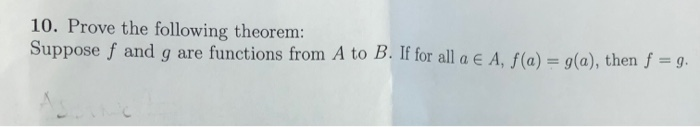10. Prove the following theorem: Suppose f and g are functions from A to B. If for all a A, f(a) g(a), then f -g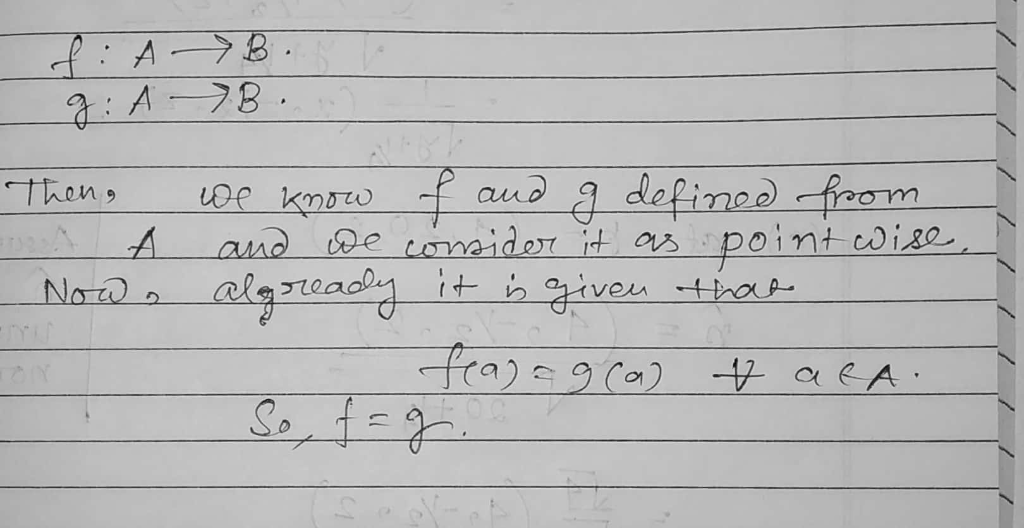If you miss any information then ask in comment section

#### Earn Coin

Coins can be redeemed for fabulous gifts.

Similar Homework Help Questions
• ### Please help me with this question，I need definition ， scratch work and proof，pls have a good...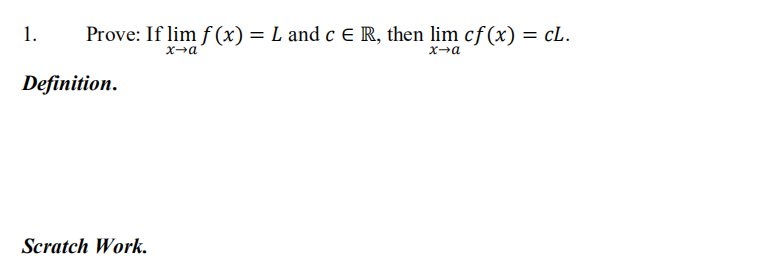Please help me with this question，I need definition ， scratch work and proof，pls have a good handwriting. Thank you so much.... 1. Prove: If lim f (x) = L and C E R, then lim cf(x) = cL. xa x-a Definition. Scratch Work. Proof:

• ### #23 22, Use the definition of limit to prove Theorem 3.5. 23. Use Theorem 3.5 to...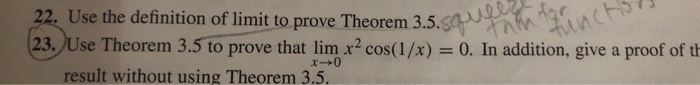#23 22, Use the definition of limit to prove Theorem 3.5. 23. Use Theorem 3.5 to prove that lim x? cost(1/x)-0. In addition, give a proof of th result without using Theorem 3.5. THEOREM 3.5 Squeeze Theorem for Functions Let I be an open interval that contains the point c and suppose that f, g, except possibly at the point c. Suppose that g(x) s f(a) s h(x) for all x in I If limn g(x)-L = lim h (x),...

• ### 10. Use the Fundamental Theorem of Calculus to provide a proof of Theorem 8.4 under the additiona...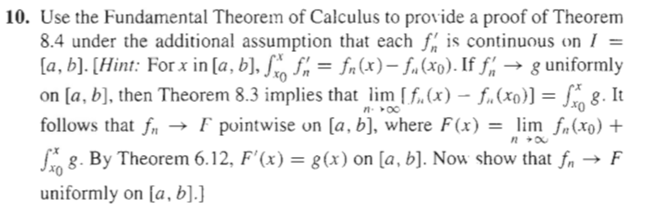10. Use the Fundamental Theorem of Calculus to provide a proof of Theorem 8.4 under the additional assumption that each fis continuous on I la, b).(Hint: For x in la, b.o)If f g uniformly on [a, b], then Theorem 8.3 implies that im f.(x) f (x8. It follows that frpuintwise on la, b), where F(x) -lim frCro) + .By Theorem 6.12, F()-x) on la,b). Now show that f uniformly on la, b].] F heorem 8.4 Suppose that neN is a...

• ### Need help figuring out this proof. Please show all work and explain if you can. 1....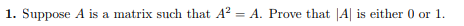Need help figuring out this proof. Please show all work and explain if you can. 1. Suppose A is a matrix such that A2 -A. Prove that A is either 0 or 1

• ### (Proof of the Squeeze Theorem for Functional Limits). Let f.g, h: A R be three functions...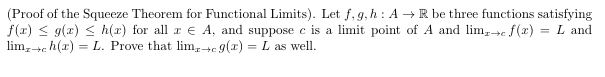(Proof of the Squeeze Theorem for Functional Limits). Let f.g, h: A R be three functions satisfying f(x) < 9(2) < h(r) for all re A, and suppose c is a limit point of A and lim; cf(x) = L and lim -ch() = L. Prove that lim.+c9(x) = L as well.

• ### 3) Complete the proof of the Pythagorean theorem: Prove: Area of Rectangle MCLE = Area of...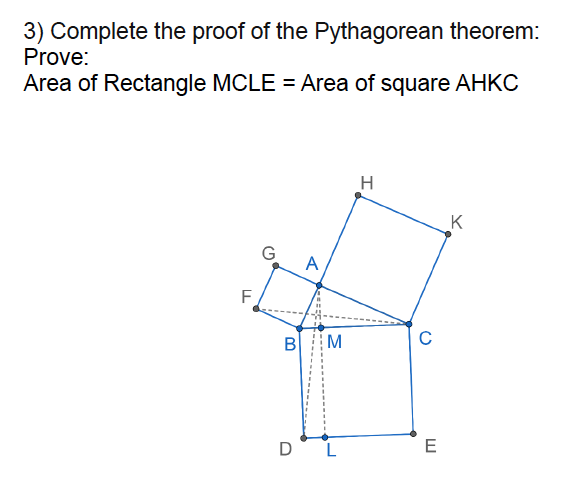3) Complete the proof of the Pythagorean theorem: Prove: Area of Rectangle MCLE = Area of square AHKC H K G A F B M C D L E

• ### Subject: Proof Writing (functions) In need of help on this proof problem, *Prove the Following:* Here...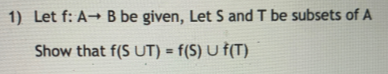Subject: Proof Writing (functions) In need of help on this proof problem, *Prove the Following:* Here are the definitions that we may need for this problem: 1) Let f: A B be given, Let S and T be subsets of A Show that f(S UT) = f(s) U f(T) Definition 1: A function f from set A to set B (denoted by f: A+B) is a set of ordered Pairs of the form (a,b) where a A and b B...

• ### F1. need help solving this problem. 1. (25 pts) Here's a neat theorem. Suppose that f la, b] [a, b] is continuous; then f will always map some s-value to itself (a so-called fixed point): i.e....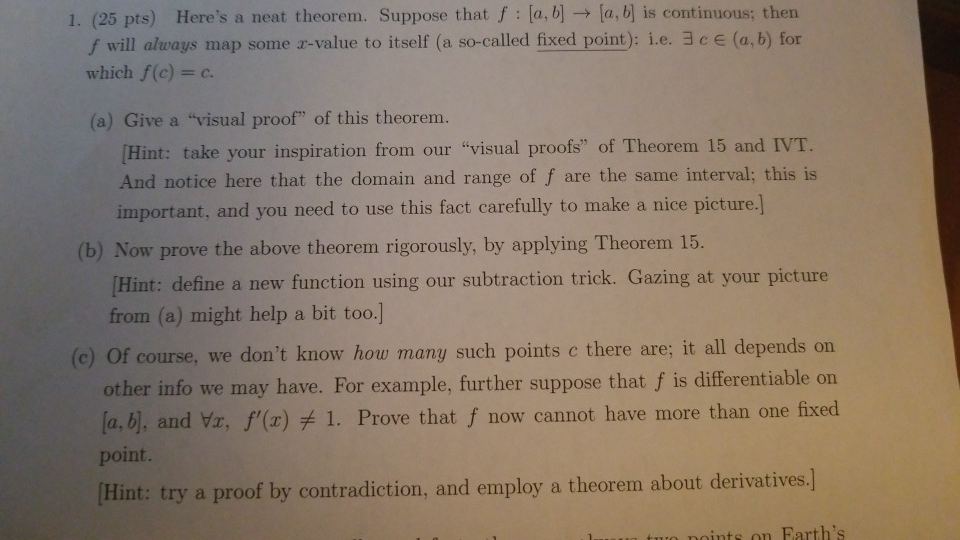F1. need help solving this problem. 1. (25 pts) Here's a neat theorem. Suppose that f la, b] [a, b] is continuous; then f will always map some s-value to itself (a so-called fixed point): i.e. 3 c E (a, b) for which f(c)-c (a) Give a "visual proof" of this theorem. Hint: take your inspiration from our "visual proofs" of Theorem 15 and IVT And notice here that the domain and range of f are the same interval; this...

• ### +Risa 3. Write down a careful proof of the following. Theorem. Let (a,b) be a possibly...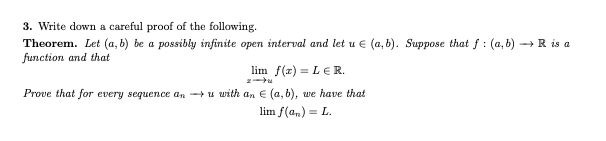+Risa 3. Write down a careful proof of the following. Theorem. Let (a,b) be a possibly infinite open interval and let u € (a,b). Suppose that f: (a,b) function and that lim f(x)=LER Prove that for every sequence an u with an E (a,b), we have that lim f(ar) = L.

• ### I need proof of this numerical analysis theorem. This theorem is from Burden's Numerical analysis book....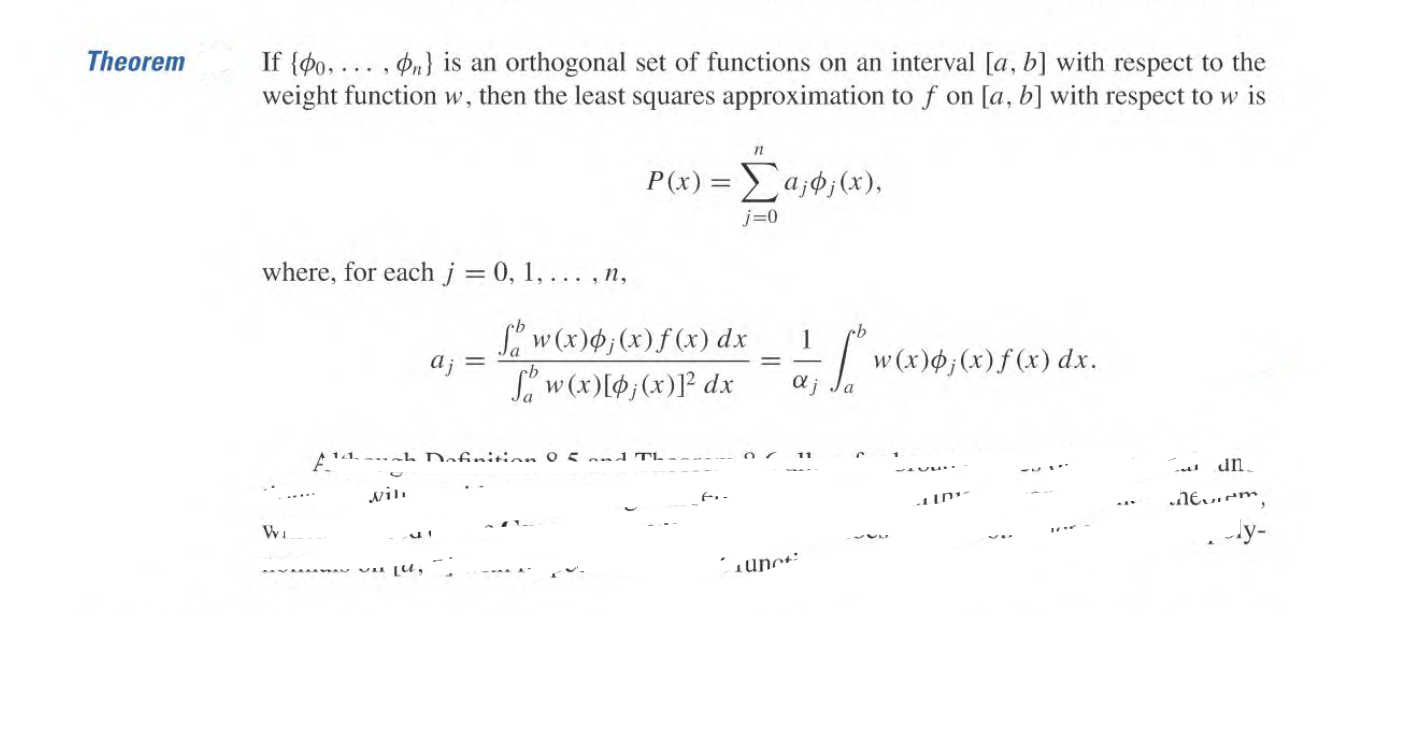I need proof of this numerical analysis theorem. This theorem is from Burden's Numerical analysis book. Please give me the detailed solution of this theorem. Theorem If {00, ... , ºn} is an orthogonal set of functions on an interval [a, b] with respect to the weight function w, then the least squares approximation to f on [a, b] with respect to w is 11 P(x) = a;°;(x), j=0 where, for each j = 0, 1, ... ,n, cb aj...

Free Homework App